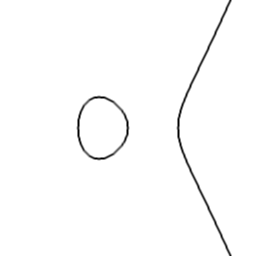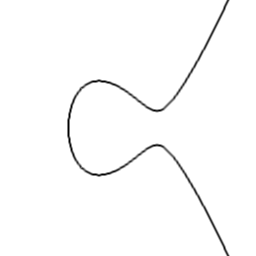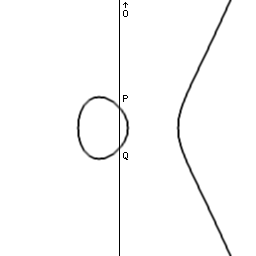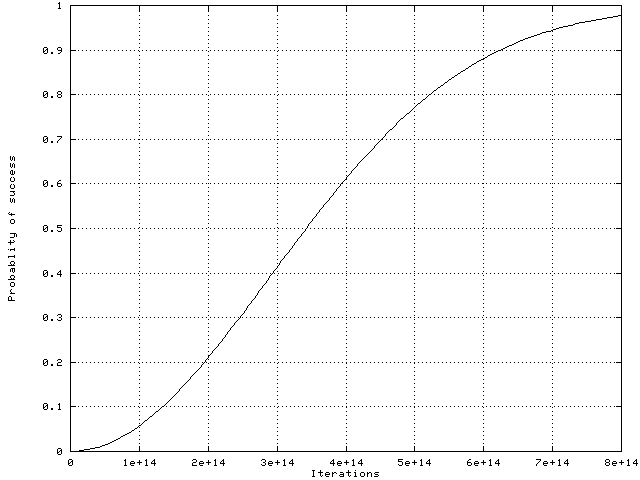# Everything you ever wanted to know about Elliptic Curve Discrete Logarithms.

## Index

1. Why is this interesting? Is it all about cracking a code?
2. What is a discrete logarithm?
3. Remind me what a finite group is.
4. What is an elliptic curve and what is the group of points on it?
5. What is a finite field?
6. What is the ECC2-97 problem?
7. What algorithm can we use to solve ECDL problems like ECC2-97?
8. What are the iterations in "iterations per second"?
9. What are distinguished points?
10. What are the initial and current expectations?
11. How hard is the ECC2-97 problem anyway?
12. How can I participate?

## 1. Why is this interesting? Is it all about cracking a code?

Discrete logarithm problems are known to be difficult. More precisely it can be shown that computing discrete logarithms takes exponential time, except when some particular extra structure is present.

In the case of elliptic curve discrete logarithms, no such extra structure is known except in a few special cases. Thus it appears that ECDLs are difficult in general.

This alone makes them very interesting for mathematician-programmers. We are constantly searching for better algorithms or implementation methods and expanding the set of special cases. Cryptographers also base the security of codes on the difficulty of computing ECDLs.

By solving ECC2-97 we are not looking for a secret message but we are looking for a secret key by solving a mathematical problem. In doing so we are also demonstrating what codes can be broken in practice.

## 2. What is a discrete logarithm?

The discrete logarithm can be defined very generally as follows.

We are given a "thing", called A, and an operation on "things", called #.

For any integer n >= 1, define [n]A to mean A # A # ... # A repeated n times.

The discrete logarithm problem is: given some B of the form [n]A, find n.

We will be concerned with the case where the "things" are in fact elements of some finite group and the operation # is the group operation. There can be several solutions n, but any one will do.

## 3. Remind me what a finite group is.

Unsurprisingly, a finite group is just a group that is finite!

An abelian group (our groups will always be abelian) is a set with an operation # satisfying these properties:

The operation is commutative: A # B = B # A.
There is a neutral element O such that A # O = A, for any A.
Each element A has an inverse I(A) such that A # I(A) = O.

The most familiar example of a group is integers with # being addition. Here O is 0 and I(A) is -A. The discrete logarithm is just division, although we know in advance that the quotient is an integer (there is no remainder).

Another example is non-zero rational numbers with # being multiplication. Here O is 1 and I(A) is 1/A. The discrete logarithm is an ordinary logarithm, except that we know that the result is an integer.

Examples of finite groups are:
- integers with addition, modulo a positive integer.
- non-zero integers with multiplication, modulo a prime integer.

We will be concerned with the case where the group is the group of points on an elliptic curve.

## 4. What is an elliptic curve and what is the group of points on it?

An elliptic curve is the set of points (x, y) satisfying an equation something like this:

y² = x³ + a·x + b
The coefficients a, b and the coordinates x, y are taken to be in some field. If it is the field of real numbers, R, then the curve can be drawn on a graph. Two examples are shown.There is a way of defining a group operation on the points. Given two points P and Q on the curve, draw a line through them and find its other intersection with the curve. Then reflect that point through the horizontal axis to find P # Q. When P = Q use the tangent line instead.Now there is one special case when there is no obvious "other intersection" and that is when P reflected through the horizontal axis is equal to Q so that the line is vertical. In this case P is the inverse of Q and so P # Q = O, the neutral group element. But where is O? Well apart from the points on the graph there is one special point called "the point at infinity", and it is O.The operation just described can be proved to satisfy the group properties. It can also be written out using rational functions in the coefficients and coordinates. Note that when the field is not R we may no longer be able to draw nice graphs but the rational functions defining the group operation still make sense and can be used to compute in the group.

We will be concerned with the case when the field is a finite field. Note that the group of points on the curve will necessarily be finite too.

## 5. What is a finite field?

It is a field that is finite, of course!

A field is a set of things with two group operations, addition and multiplication, written + and ·.

The field elements must form an (abelian) group under addition and the non-zero ones must form an (abelian) group under multiplication. Furthermore there is a distributive law like this:

a·(b+c) = a·b + a·c.
Typical examples are rational numbers, real numbers or complex numbers.

An example of a finite field is the set of integers modulo a prime integer. The simplest possible one is the integers modulo two i.e., the booleans. Addition and multiplication are XOR and AND respectively. You can check all the field properties if you like!

0 + 0 = 0
0 + 1 = 1
1 + 0 = 1
1 + 1 = 0

0 · 0 = 0
0 · 1 = 0
1 · 0 = 0
1 · 1 = 1

One way to get a large finite field is to take a large prime. Another way is to take a small prime, such as 2, and do a field extension.

For example, start with the boolean field just described. Now we can write polynomials (in one variable) with coefficients in the boolean field. Here is one:

t^6 + t³ + t² + t + 1
These polynomials can usually be factored. In this case:
(t² + t + 1) · (t^4 + t³ + 1)
=
t^6 + 2·t^5 + 2·t^4 + t³ + t² + t + 1
=
t^6 + t³ + t² + t + 1
(modulo 2)
When the polynomial has no factors other than itself or 1, we say that it is a prime polynomial. Here is one:
t^97 + t^6 + 1
Now we can do arithmetic on polynomials modulo this prime polynomial in roughly the same way as we do arithmetic on integers modulo a prime integer. In this way we get a field with 2^97 elements. This is the field used for the ECC2-97 problem.

## 6. What is the ECC2-97 problem?

It is an ECDL problem, defined by Certicom, as follows.

Take the field with 2^97 elements. The elliptic curve is:

y² + x·y = x³ + a·x² + b
where the coefficients are:
a = 151759946635783345666111607832
b =  46586505053717239594563606920
The points P and Q for which we have to find n with [n]P = Q are:
P = (46059744771359721362870909225, 98068469436517043337972206208)
Q = (72978944960364674557961530030, 96375666913718821189526238111)
Note that these are really boolean polynomials modulo t^97 + t^6 + 1 but have been written as integers by setting t to 2.

The group of points on this curve has roughly 2^97 points (it is always the case that the group size is close to the finite field size). However the ECC2-97 problem takes place in the sub-group of half the size i.e., roughly 2^96 points. The precise number is:

g = 79228162514264464603828067969

## 7. What algorithm can we use to solve ECDL problems like ECC2-97?

For computing discrete logarithms in small groups, it is possible to do an exhaustive search of all possible solutions until one works. This method is useless though for a group with close to a hundred billion billion billion elements.

A much smarter way is to use the birthday paradox. It says that if you pick 23 people, then there is more than a 50 % chance that two of them have the same birthday. The reason why only 23 people are needed is that the number of pairs of people grows in proportion to the square of the number of people.

It is the same thing with points on an elliptic curve! If we compute "only" about four hundred thousand billion random points, then chances are good that two will in fact be the same.

We compute points of this form: [s]P # [t]Q with random s and t. If two are the same then:

[s]P # [t]Q = [u]P # [v]Q
so:
[s-u]P = [v-t]Q
so:
[(s-u)/(v-t)]P = Q
So the answer n is (s-u)/(v-t), computed modulo the group size g.

## 8. What are the iterations in "iterations per second"?

To compute points [s]P # [t]Q with random s and t we do not pick new s and t values every time. Computing [s]P # [t]Q from scratch every time would be too slow. Instead we change s and t a bit each time.

For instance if we are at a random point R = [s]P # [t]Q, then we can jump to R # R = [2s]P # [2t]Q with a single elliptic operation.

Likewise if we pre-computed a constant point C = [c]P and we are at a random point R = [s]P # [t]Q, then we can compute R # C = [s+c]P # [t]Q with a single elliptic operation.

We actually use both of these: we either double, or add one of 15 different constant amounts at each step. The choice is made using a pseudo-random hash function.

If you run the ecdl2-97.c program and it reports something like 123456 iterations per second, that means that it is jumping from point to point by doing these adding-or-doubling steps 123456 times every second.

Clearly it would be impossible to send all the points back to base to check for a match between two points. That is why we have distinguished points.

## 9. What are distinguished points?

Distinguished points are those rare random points that happen to have a distinguishing feature, namely they have a bunch of 30 zero bits in the middle of their y coordinates. The particular feature does not matter much but it must be fixed in advance. This feature occurs one time in 2³° on average (roughly once per billion).

Note that depending on chance it can sometimes take half a billion or three billion iterations to find a distinguished point. Don't worry about being "out of luck" though because all points are not quite equal: the value of a point is proportional to the number of iterations it took!

An important thing to notice is that everybody is using the same pseudo-random way of stepping from one random point to another.

When somebody eventually finds a random point which is the same as one already visited, then they will follow the exact same adding-or-doubling steps as the first visitor did, until after a while they find a distinguished point and send it in.

That distinguished point was already found and sent in by the first visitor, so back at base we find the match between distinguished points and compute the answer (s-u)/(v-t) as described above.

## 10. What are the initial and current expectations?

We need to do a total of four hundred thousand billion steps to visit four hundred thousand billion random points. One per billion of those is distinguished so we expect four hundred thousand distinguished points.

The reason that the current expectation is a bit larger than the initial expectation is similar to what happens when doing an exhaustive search. At first you expect to examine 50 % of the search space. But if you have already checked 20 % without success, you then expect to have to go to 60 % instead of 50. We are not doing an exhaustive search but the idea is similar.

Depending on luck, we could find a match after three hundred thousand or it might take five but there is no way to tell in advance. This graph shows how our probability of success rises with the number of iterations.## 11. How hard is the ECC2-97 problem anyway?

If things work out as expected then the total amount of work will be something like 40000 or 50000 MIPS-years which is twice as much as ECCp-97 took and five times as much as the recently completed factorisation of RSA-155.

I believe it is accurate to say:

This is the largest public calculation ever to use a complex parallel algorithm.
where "complex parallel algorithm" is understood to exclude trivially parallel exhaustive searches. (If you disagree with this statement, please let me know by email: Robert.Harley@inria.fr).

There have been a few bigger distributed calculations. For instance a DES crack by exhaustive search is 5 to 10 times bigger, but no complex parallel algorithm is required.

In such a case, coordination is not necessary so it doesn't matter much whether one group organises the whole lot or ten groups each organise separate smaller calculations using different software (and dedicated hardware!) Some work may be duplicated but that causes only a small constant-factor slowdown.

In the end, one lucky individual finds the DES key. Likewise with RC5 cracking. Same thing with finding a new Mersenne prime or a SETI@home block with a message from aliens.

However large factorisations and discrete logarithm calculations are more complex. No single person finds the answer. Ten teams each doing ten times less work would have little hope of success.

With this ECDL project, the answer can only be found because we are all working together using the same distinguishing feature, the same pseudo-random hash function and because we are comparing everybody's distinguished points with everyone else's.

When starting out I wondered if this project wouldn't turn out to be a case of "biting off more than you can chew". But the response so far has been phenomenal and it looks like we'll make it. Even if for some reason we don't manage, consider this:

The credit belongs to the man in the arena whose face is marred by dust and sweat and blood, who strives valiantly, who errs, and who comes up short again and again, who knows the great enthusiasms, the great devotions, and spends himself in a worthy cause. The man who at best knows the triumph of high achievement and who at worst, if he fails, fails while daring greatly, so that his place will never be with those cold timid souls who never knew victory or defeat.
- Theodore Roosevelt